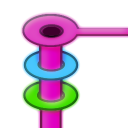#The flattening algorithm

## What is flattening and why it is needed

This is a core part of ZofzPCB concept – handling the pcb drawing as “vectors”, all way from ECAD data, down to the graphic engine. The GPU handles a list of triangles, with extreme speed, but the data needs to be converted first.

The input side is a collection of pads, tracks, or general polygons. The ECAD concept, historically, starts from a Gerber photo-plotter – the elements can overlap, in a simple way (like consecutive segments of tracks) or in an arbitrary way, called “painting”. Next development step, was a use of a negative film, allowing to construct ground or power planes, where negative disks have been blocking a plane connection to selected vias or THT-pads. The negative tracks allowed creation of power plane divisions. The last step, introduction of raster-scan photoplotters, have allowed removal of the already plotted surfaces, in pixel image memory, making possible to use positive and negative drawing style, simultaneously, on a single page.

For ZofzPCB it boils down to:

1. converting all drawing elements to edge-defined polygons (line or arc edges),
2. performing Boolean operations (positive and negative drawing),
3. Removing arcs (arc to fan conversion)
4. Applying effects and triangulation (converting polygons to set of triangles)

In addition, to know the size of the universe (e.g. negate of 0) ZofzPCB must convert the whole board drawing (made from tracks) into a polygon.

To accomplish the tasks, there is a need to determine crossing points of overlapping polygons, i.e. crossing points of line segments and arcs, several times in the process. This seems to be a very simple task, but it is not:

1. Speed
2. Round-off error and Numerical Stability

## Speed

To check for crossing points, first by a naïve concept, the program needs to check every pair of edge segments. If there is m + n edges of 2 polygons, the program needs to check (m*n)/2 pairs. The sped of the algorithm is noted O(n2) - meaning it is proportional to the square of number of segments. This does not look particularly scary, but the complication of the shapes makes it a very high number of operations. Using, so called, bounding boxes, helps, but only in some cases.

Using a “scan line” algorithm, luckily, brings a spectacular improvement. The number of tests drops down to O(n log(n)). It is a similar concept to a successive approximation ADC, as an improvement of a Integrating ADC, especially if you notice that log(n) is proportional to a number of bits representing number n.

I have already implemented this algorithm, but only on the part after removing the arc-edges. You can see the difference – triangulation is much faster then flattening, especially for more complex designs. The original algorithm does not work for arcs. Now, I have developed the modification of the algorithm, fitting the other need. I sill need too implement it, however.

## Stability

Using floating point numbers, is, by design, inaccurate. Geometrical algorithms do not account for inaccurate calculations. This is especially important when calculating circular shapes, as the square root have no defined accuracy. (Contrary, the 4 simple arithmetic operations, even if not accurate, are clearly defined, on the bit level.) The reasonable solution, turns out to be a double-double library, allowing of roughly doubling the calculation precision, on the cost of execution time. Therefore, increasing the stability, this way, would be simply killing already slow flattening speed. That means the speed improvement must be made first.

## The 3-state geometrical resolution

“The geometry is about making decisions”

The flattening algorithm uses 3 possible results of geometrical resolutions: yes, no, do-not-know. Example: when ordering edges passing via a single point and two edges have a very similar direction. The not-know state is handled by building a Boolean equation, usually resulting in a simple Boolean equation system, to be solved. Typically, the equation system is solved immediately, because it is simple. (Solving time is O(n3)) But, sometimes, the equation system is underdetermined. Then, the solution is found, by running all possible combinations, that is O(2n), and that is, of course, a misfortune. The error calculation might also be wrong, then the discrepancies occurs and the program crashes.

## Conclusion

When the more exact arithmetic will be used, the third state occurrence should be virtually removed. That is, reduced by a factor of about 240. This will reduce the probability of error even more, as the errors are happening in a complex configuration of the third states.